# Least common multiple

(diff) ← Older revision | Latest revision (diff) | Newer revision → (diff)

The smallest positive number among the common multiples of a finite set of integers or, in particular, of natural numbers,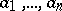. The least common multiple of the numbersexists if. It is usually denoted by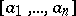.

Properties of the least common multiple are:

1) the least common multiple ofis a divisor of any other common multiple;

2)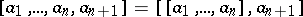;

3) if the integersare expressed as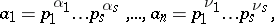where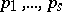are distinct primes,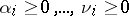,, and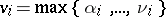,, then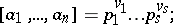4) if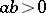, then, where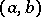is the greatest common divisor ofand.

Thanks to the last property, the least common multiple of two numbers can be found with the aid of the Euclidean algorithm. The concept of the least common multiple can be defined for elements of an integral domain, and also for ideals of a commutative ring.

How to Cite This Entry:
Least common multiple. Encyclopedia of Mathematics. URL: http://encyclopediaofmath.org/index.php?title=Least_common_multiple&oldid=17219
This article was adapted from an original article by V.I. NechaevA.A. Bukhshtab (originator), which appeared in Encyclopedia of Mathematics - ISBN 1402006098. See original article